# Analysis Of JEE Main June '22 Maths Paper

###### BySafeer PP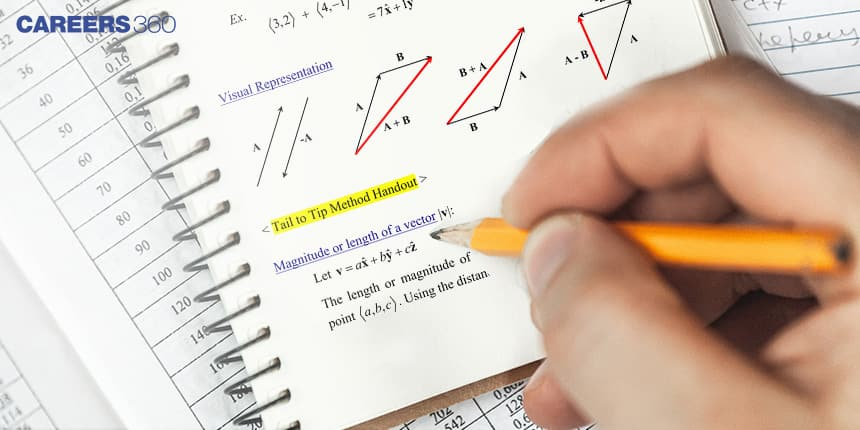###### Synopsis

JEE aspirants are extremely competitive when it comes to scoring well in Mathematics. Hence it is important to know what was asked in previous papers of JEE Main.  Let us see the  analysis of Mathematics part of JEE Main Paper 1 of the June session.###### Synopsis

JEE aspirants are extremely competitive when it comes to scoring well in Mathematics. Hence it is important to know what was asked in previous papers of JEE Main.  Let us see the  analysis of Mathematics part of JEE Main Paper 1 of the June session.

The Joint Entrance Examination (JEE) Main is conducted every year for admissions to various engineering colleges across India. JEE Main has two papers. Paper 1 is for admission to BTech/BE programmes and Paper 2 is for admission to BArch and B. Planning courses. The Paper 1 has questions from three subjects Physics, Chemistry and Mathematics with an equal number of questions from each subject. Careers360’s experts analysed the Mathematics section of JEE Main June 2022 papers. An analysis of 360 questions from 12 papers of JEE Main 2022 June session are given below along with the chapters and number of questions.

### Chapter Wise Number Of Questions

 Chapter Unit Number Of Questions Weightage(%) Co-Ordinate Geometry Geometry 45 12.50% Limit , Continuity And Differentiability Calculus 44 12.22% Integral Calculus Calculus 35 9.72% Complex Numbers And Quadratic Equations Algebra 26 7.22% Matrices And Determinants Algebra 25 6.94% Trigonometry Trigonometry 25 6.94% Three Dimensional Geometry 3-D And Vectors 24 6.67% Differential Equations Calculus 23 6.39% Permutations And Combinations Algebra 19 5.28% Sequence And Series Algebra 18 5.00% Statistics And Probability Algebra 18 5.00% Sets, Relations And Functions Calculus 17 4.72% Binomial Theorem And Its Simple Applications Algebra 15 4.17% Vector Algebra 3-D And Vectors 15 4.17% Mathematical Reasoning Algebra 11 3.06%

Coordinate Geometry, Limits Continuity And Differentiability and Integral Calculus have a weightage of 12.5 per cent, 12.22 per cent and 9.72 per cent respectively in JEE Main 2022 June session. These three units had the highest weightage of 11.4 per cent 10.38 per cent and 9.74 per cent respectively in the 2021 JEE Main Mathematics paper. Calculus and Algebra covered more than 60 per cent of the paper.

### Mathematics: Ten Most Asked Concepts JEE Main 2022 Session 1 Paper 1

Linear Differential Equation has 13 questions in the June 2022 JEE Main paper and the same topic has 19 questions including both sessions of the 2021 JEE Main paper. The other important topics asked are Cramer's Rule and Area Bounded By Two Curves.

### 10 Most Asked Concepts JEE Main 2022 Session 1

 Topic Number OfQuestions Linear Differential Equation 13 Cramer’s Law 10 Area Bounded By Two Curves 10 Vector (Or Cross) Product Of Two Vectors 9 Composition Of Function, Condition For Composite Function,Property Of Composite Function 7 General Term Of Binomial Expansion 7 Trigonometric Equations 7 Multiplication Of Two Matrices 6 Family Of Plane 6 Dispersion (Variance And Standard Deviation) 6

From the above analysis, students can plan the areas to be concentrated while preparing for JEE Main Mathematics. Questions from all the units are covered with a minimum weightage of 3.06 per cent and a maximum of 12.5 per cent. This analysis shows that all the chapters given in the JEE Main syllabus are important for preparation. It is to be noted that Mathematics is a subject that requires a high amount of practice. Based on the weightage of the chapter, students preparing for JEE Main can make their time table.

• Calculus
• Joint Entrance Examination
• Joint Entrance Examination – Main
• Mathematics
• Mathematical objects
• Mathematical concepts
• Mathematical analysis
• Equation
• Euclidean vector
• Function (mathematics)

## Careers360 helping shape your Career for a better tomorrow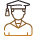#### 250M+

Students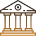#### 30,000+

Colleges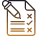#### 500+

Exams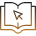E-Books